﻿ Electronic Circuit Simulation of the Lorenz Model With General Circulation

### Electronic Circuit Simulation of the Lorenz Model With General Circulation

D. C. Saha, Anirban Ray, A. Roy ChowdhuryOPEN ACCESSPEER-REVIEWED

## Electronic Circuit Simulation of the Lorenz Model With General Circulation

D. C. Saha1, Anirban Ray2, A. Roy Chowdhury2,1Department of Physics, Prabhu Jagatbandhu College, Andul Howrah, India

2High Energy Physics Division, Department of Physics, Jadavpur University, Kolkata, India

### Abstract

The nonlinear dynamics of the Lorenz model of general circulation is investigated with the help of analogue electronic circuits. The structure of the attractor is obtained for the various values of the systems parameters. Existence of two external potential terms in the equation leads to some new and interesting features. The data so generated is collected through the use of NI-6009 USB, analogue to digital converter. This was then used to compute the bifurcation pattern, parametric Lyapunov diagrams, Lyapunov exponents. The system clearly showed a non-periodic doubling route to chaos. This is farther substantiated by the simple variation of Lyapunov exponent in bi-parametric space of forcing parameter for the system. These external forcing is actually very important to settle the various issue arising in the long time behavior.

### At a glance: Figures

123
Prev Next

• Saha, D. C., Anirban Ray, and A. Roy Chowdhury. "Electronic Circuit Simulation of the Lorenz Model With General Circulation." International Journal of Physics 2.5 (2014): 124-128.
• Saha, D. C. , Ray, A. , & Chowdhury, A. R. (2014). Electronic Circuit Simulation of the Lorenz Model With General Circulation. International Journal of Physics, 2(5), 124-128.
• Saha, D. C., Anirban Ray, and A. Roy Chowdhury. "Electronic Circuit Simulation of the Lorenz Model With General Circulation." International Journal of Physics 2, no. 5 (2014): 124-128.

 Import into BibTeX Import into EndNote Import into RefMan Import into RefWorks

### 1. Introduction

Analysis of non-linear dynamical systems from the view point of stability is a well known field of theoretical research, which has gained momentum over the last few decades . The analogue electronic circuits can be used to simulate a nonlinear system of ode’s and resulting output can be explicitly visualized on an oscilloscope screen. This approach got a boosting with the advent of Chua’s circuit [2, 3, 4]. He was the first one to predict an electronic circuit whose output is to be analyzed with nonlinear dynamics. After that Carroll and Pecora [5, 6, 7] used circuit implementation of R¨osseler system to simulate chaos control and synchronization in real life. Another successful application of this method was its use on the original Lorenz equation in describing chaos synchronization and control. Such an application was initially put forwarded by Cuomo and Oppenheim [8, 9]. Later such methods were efficiently used to synchronize different non-linear dynamical systems in communication purposes  and such methods were also efficiently used to designs chaotic cryptosystems [11, 12]. In this communication we have studied a new form of Lorenz equation [13, 14, 15], (long-term atmospheric circulation) with the help of electronic circuit. The pattern of the attractor formation was displayed through the oscilloscope. But for the details of the properties associated with the attractor, the data was collected by an analog to digital converter (USB NI-6009). These data were then studied for the analysis of the Lyapunov exponents, bifurcation structure and shrimp structure [16, 17] or the existence of periodic islands in the chaotic sea.

### 2. Formulation

The new Lorenz model which takes care of general circulation was analyzed theoretically by Masoller et al [18, 19, 20]. This model proposed by Lorenz in 1984 is a Galerkin truncation of the Navier- Stokes equations and gives the simplest approximation to the general atmospheric circulation at mid-altitude. The equation can be written as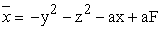(1a)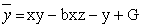(1b)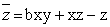(1c)

where the variable x represents the strength of a large scale western wind current, while y and z are the strengths of the cosine and sine phases of a chain of superposed waves. The parameter F represents the external heating contrast between oceans and continents. In the following we shall explore a variety of values of these parameters (F, G), which will show the existence of three different type of attractors.

Figure 1. Schematics of the electronic circuit explore a variety of values of these parameters (F, G), which will show the existence of three different type of attractors

The electronic circuit pertaining to Eq. (1) is shown in Figure 2. Due to various non-linear terms, we have four multipliers A1, A2, A3 and A4 all are IC’s of type AD633, whereas U1, U2, U3, U4 are standard opamps AD741. The resistances and capacitance are all identified in the circuit with their values shown. In the figure F, G represents two external potentials. The physical situation considered uses the parameter values a = 1/4, b = 4, G = 1 and F takes up three values 4.56, 5.198 and 8.0.

In the following, we give a step by step account for obtaining the circuit shown in Figure 2. To begin with we start with the integrator circuit for x (Figure 2).

Corresponding equation is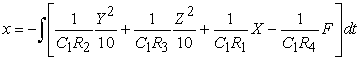(2)

which is to be compared with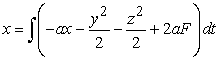(3)

Now scaling the variable by a factor of 2, that is x→x/2, y→y/2 and z→z/2. If we compare them we can assign suitable values or the resistance and capacitance.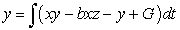(4)

This is shown in Figure 2. Comparing Eq. (4) with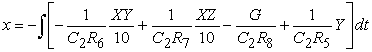(5)

And for the third equation we use,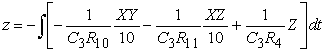(6)

which is to be compared to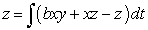(7)

Corresponding circuit is shown in Figure 2. In all of the above circuits the products xy, xz, y2, z2 are to constructed with the help of multipliers AD633. Collecting all these together we get the circuit in Figure 1 and simultaneously the values of parameter can be fixed.

The experimental data collected in the form of the three vectors x, y and z, are then utilized to draw the various situations in the phase space. In the Figure 5a to 5h we show for each case of parameters values both the figure obtained from such data structure and also the same form as observed on the oscilloscope screen. The similarity is really very striking. These data is then used to compute the Lyapunov coefficients and a graphical representation of such analysis is shown in Figure 6(a). To vary G and F, we employed different weir-wound potentiometer combinations. On the other hand the bifurcation diagram with respect to G is given in Figure 6(a). From this figure it appears that there are several stages of the creation and destruction of attractors as G is varied.

The scenario is also supported by the computation of Lyapunov exponents as given in Figure 7(a).

A similar events also takes place if we consider variation of F. These are reported in Figure 6(b) and Figure 7(b). Variation of Lyapunov exponent in bi-parametric plane is given in Figure 8. This diagram shows chaotic regions along with periodic regions. Here, variation of yellow to red color represent different chaotic region and variation from blue to green color represent the periodic regions. As can be seen from close observation of the figure, this chaotic regions sometimes form geometric structure like ‘shrimp’. For each parameter value, 10000 data were collected for computing maximum lyapunov exponents on each stage. After that, we calculated the template structure of the present system.

For this, we followed the procedure represented in Ref. . First, we extracted the UPO’s (unstable periodic orbits) from the chaotic attractor at parameter value a = 0.25, b = 4.0, F = 8.0, G =1.149. Then we have calculated the template structure of the attractor. Template structure is the two dimensional embedding of the attractor, keeping all its integration between UPO’s intact. This is described with the following matrix.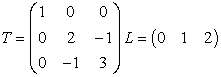Here ‘T’ is the template matrix and ‘L’ is the layering matrix. Its graphical representation is shown in Figure 2. Here arrowhead represents the direction of the flow. Additionally, ‘0’, ‘1’ and ‘2’ represent three different period one orbits.

### 3 Conclusion

In our above analysis we have discussed the chaotic aspect of a atmospheric model from the point of view of electronic circuits. We first fabricated the circuit then went on to collect the data through a ADC converter. We have studied bifurcation of the system along two parameters G and F. We also calculated the variation of maximum lyapunov exponents from the data and calculated the variation of maximum lyapunov exponents with two parameters G and F. Latter this is shown in detail in the bi-parametric diagram on G vs. F plane.

Figure 6. (a) Bifurcation with variation of parameter G while F = 8.0. (b) Bifurcation with variation of parameter F while G = 1.0
Figure 7. (a) Maximum lyapunov exponent with variation of parameter G while F = 8.0. (b) Maximum lyapunov exponent with variation of parameter F while G = 1.0

### References

  S. H. Strogatz, Nonlinear Dynamics And Chaos: With Applications To Physics, Biology, Chemistry, And Engineering (Studies in Nonlinearity), 1st Edition, Studies in nonlinearity, Westview Press, 2001.In article  T.Matsumoto, A chaotic attractor from chua’s circuit, Circuits and Systems, IEEE Transactions on 31 (12) (1984) 1055-1058.In article  L. O. CHUA, Chua’s circuit: An overview ten years later, Journal of Circuits, Systems and Computers 04 (02) (1994) 117-159.In article CrossRef  R. Madan, Chua’s Circuit: A Paradigm for Chaos, Journal of circuits, systems, and computers, World Scientific, 1993.In article  T. Carroll, L. M. Pecora, Synchronizing chaotic circuits, Circuits and Systems, IEEE Transactions on 38 (4) (1991) 453-456.In article  L. M. Pecora, T. L. Carroll, Driving systems with chaotic signals, Phys. Rev. A 44 (1991) 2374-2383. URL http://link.aps.org/doi/10.1103/PhysRevA.44.2374In article CrossRef  L. M. Pecora, T. L. Carroll, Synchronization in chaotic systems, Phys. Rev. Lett. 64 (1990) 821-824. URL http://link.aps.org/doi/10.1103/PhysRevLett.64.821In article CrossRef  K. Cuomo, A. Oppenheim, S. H. Strogatz, Synchronization of lorenz-based chaotic circuits with applications to communications, Circuits and Systems II: Analog and Digital Signal Processing, IEEE Transactions on 40 (10) (1993) 626-633.In article  K. M. Cuomo, A. V. Oppenheim, Circuit implementation of synchronized chaos with applications to communications, Phys. Rev. Lett. 71 (1993) 65-68. URL http://link.aps.org/doi/10.1103/PhysRevLett.71.65In article CrossRef  E. M. Bollt, Review of chaos communication by feedback control of symbolic dynamics, International Journal of Bifurcation and Chaos 13 (02) (2003) 269-285.In article CrossRef  E. S´anchez, M. A. Mat´ıas, Experimental observation of a periodic rotating wave in rings of unidirectionally coupled analog lorenz oscillators, Phys. Rev. E 57 (1998) 6184-6186. URL http://link.aps.org/doi/10.1103/PhysRevE.57.6184In article CrossRef  O. Gonzales, G. Han, J. de Gyvez, E. Sinencio, Lorenz-based chaotic cryptosystem: a monolithic implementation, Circuits and Systems I: Fundamental Theory and Applications, IEEE Transactions on 47 (8) (2000) 1243-1247.In article  E. N. Lorenz, Irregularity: a fundamental property of the atmosphere*, Tellus A 36A (2) (1984) 98-110. URL http://dx.doi.org/10.1111/j.1600-0870.1984.tb00230.xIn article  H. Broer, C. S. A, R. Vitolo, Bifurcations and strange attractors in the lorenz-84 climate model with seasonal forcing, Nonlinearity 15 (4) (2002) 1205.In article CrossRef  L. van Veen, Baroclinic flow and the lorenz-84 model, International Journal of Bifurcation and Chaos 13 (08) (2003) 2117-2139.In article CrossRef  E. N. Lorenz, Compound windows of the hnon-map, Physica D: Nonlinear Phenomena 237 (13) (2008) 1689-1704. URL http://www.sciencedirect.com/science/article/pii/S0167278907004198.In article CrossRef  J. A. C. Gallas, Structure of the parameter space of the h´enon map, Phys. Rev. Lett. 70 (1993) 2714-2717. URL http://link.aps.org/doi/10.1103/PhysRevLett.70.2714In article CrossRef  C. Masoller, A. Schifino, L. Romanelli, Regular and chaotic behavior in the new lorenz system, Physics Letters A 167 (2) (1992) 185-190.In article CrossRef  C. Masoller, A. S. Schifino, L. Romanelli, Characterization of strange attractors of lorenz model of general circulation of the atmosphere, Chaos, Solitons & Fractals 6 (0) (1995) 357-366, complex Systems in Computational Physics.In article CrossRef  A. Schifino, C. Masoller, Analitical study of the codimension two bifurcations of the new Lorenz system, in: E. Tirapegui,W. Zeller (Eds.), Instabilities and Nonequilibrium Structures V, Vol. 1 of Nonlinear Phenomena and Complex Systems, Springer Netherlands, 1996, pp. 345-348.In article  R. Gilmore, M. Lefranc, The Topology of Chaos: Alice in Stretch and Squeezeland, Wiley, 2008. URL http://books.google.co.in/books?id=XuXDrtthXHcCIn article